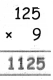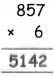Students can download 4th Maths Term 2 Chapter 2 Numbers Ex 2.1 Questions and Answers, Notes, Samacheer Kalvi 4th Maths Guide Pdf helps you to revise the complete Tamilnadu State Board New Syllabus, helps students complete homework assignments and to score high marks in board exams.

## Tamilnadu Samacheer Kalvi 4th Maths Solutions Term 2 Chapter 2 Numbers Ex 2.1

Question 1.
Multiply the following numbers by using lattice method.
(i) 52 × 49
(ii) 347 × 5
(iii) 127 × 7
(iv) 65 × 37
(v) 789 × 4
(vii) 37 × 29
(i) 52 × 49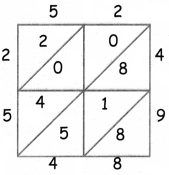52 × 49 = 2548(ii) 347 × 5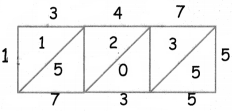347 × 5 = 1735

(iii) 127 × 7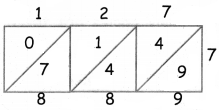127 × 7 =889

(iv) 65 × 37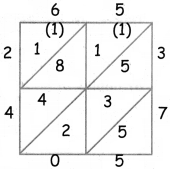65 × 37 = 2405(v) 789 × 4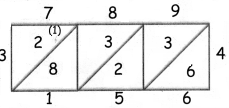789 × 4 = 3156

(vii) 37 × 29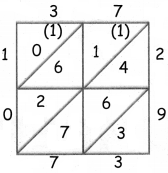37 × 29 = 1073Question 2.
Multiply the following numbers by using standard method
(i) 72 × 29
(ii) 31 × 27
(iii) 93 × 42
(iv) 124 × 6
(y) 206 × 8
(vi) 479 × 3
(i) 72 × 29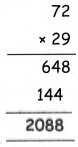72 × 29 = 2088

(ii) 31 × 27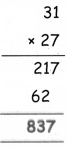31 × 27 = 837(iii) 93 × 42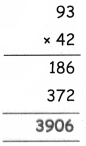93 × 42 = 3096

(iv) 124 × 6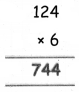124 × 6 = 744(v) 206 × 8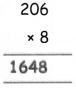206 × 8 = 1648

(vi) 479 × 3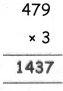479 × 3 = 1437Question 3.
One box contains 25 apples. How many apples are there in 36 such boxes?
No. of apples in a box = 25
No. of apples in 36 boxes = 25 × 36
= 900 apples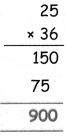i. There are 28 pages in a daily newspaper. If Ajay buys newspapers for 45 days. how many pages will it contain?
No. of pages in a daily newspaper = 28
No. of pages in 45 days = 28 × 45
= 1260 Pages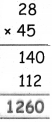ii. A truck carries 125 bags of rice. [f each bag has 9 kg of rice0 how much kg of rice will there be in the truck,